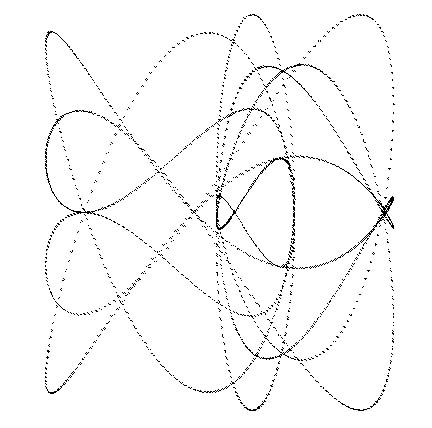### ‘back to basics’ harmonograph

After yesterday’s unsatisfying complexity, I decided to start afresh.

I started with the basic formula for a circle: x = r*sin(theta) and y=r*cos(rho), where r is the radius of the desired circle and theta start starts at 0.0 and is gradually incremented, to provide each point on the circle’s circumference. I gave theta a different to calculate x and y, and added a different weight to each of these formulae, to become x = r*sin(thetaX) + sin(Wx) and y = r*sin(thetaY) + sin(Wy). Note that at this stage, unlike theta, Wx doesn’t vary over time, nor is there a damping effect. This produced the following simple image:The pen started to repeat itself very quickly, going over exactly the same lines.

Tags: ,

Saturday, November 16th, 2013 coding